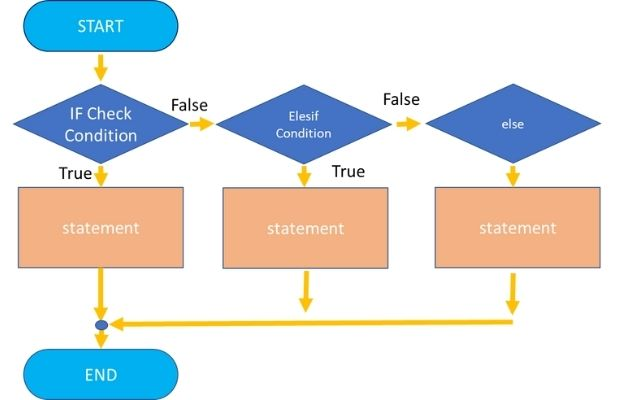# PHP IF ELSE statementPHP IF statement is popular and is used to check some conditions and accordingly statements are executed. We can control the html display part also by the IF STATEMENT. Here is one simple example of if statement.
``````\$j = 5;\$k = 10;
if (\$j < \$k){print "Variable \$j value is less  than \$k";
}``````
We can add ELSE condition to the above statement and that part will be executed once the if condition does not satisfy.
``````\$j = 10;\$k = 5;
if (\$j < \$k){print "Variable \$j value is less  than \$k";
// This line will not be executed
}
else{
print "Variable \$j value is greater  than \$k";
// This line will be executed
}``````

If we are checking the different values of a variable which can have more than two conditions then for each case using PHP IF STATEMENT for matching conditions  is not a good idea. In such a case  PHP SWITCH STATEMENT  is to be used.

PHP if else and elseif with ternary operators to check conditions and execute statement blocks

## elseif

Instead of using else we can use elseif to check more conditions. Here is another example
``````\$j=8;
\$k=5;
if(\$j<\$k){
echo " Variable \\$j \$j value is less than \\$k \$k";
}elseif(\$j>10){
echo " Variable \\$j \$j value is greater than 10";
}else{
echo " variable \\$j \$j is less than 10 but greater than 5";
}``````
Output is
``variable \$j 8 is less than 10 but greater than 5``
Once all the conditions are False the last line is executed.
By changing the variable \$j value to 12 , we will get this output
``Variable \$j 12 value is greater than 10``

## Using Ternary operators

Instead of wring if .. else conditions we can use ternary operator ( ? ) to reduce the codes. This is short cut to writing if else statements.
``````\$mark=65;
\$status=\$mark > 50 ? 'Pass':'Fail';
echo \$status; # output is Pass ``````
We can receive name from Query string and use the same inside our script.
``````\$name=strlen(\$_GET['name'])>2 ? \$_GET['name'] : 'anonymous';
print(\$name);``````

## Nested if condition

Inside one if condition check we can keep additional checks also.
``````\$mark=80;
if(\$mark >= 50){
echo " You Passed, ";
if (\$mark >=80){
echo " You got Distinction also";
} // end of inner if condition
}// end of outer if condition``````

## Switch and multiple if else

When to use switch and when to use if condition checking in PHP scripts ? Both are available to use but they are to be used at appropriate place. Here are some examples.

Day of the week and matching code.
Here based on the day of the week we have to execute matching code block. Better to use switch condition as we know one out of 7 choices available will match.

Deciding pass or fail based on the mark secured by student in a exam
If mark is less than 50 then outcome is fail and if mark is equal to or more than 50 then pass. Here better to use IF condition.

Deciding grade based on the mark
Better to use switch condition as based on different ranges of mark we can decide the grade of the student. Say 50 to 79 is grade C, 80 to 89 grade B and 90 above grade A.

Checking the session of user is logged in or not
Use if condition.
``````if (isset(\$_SESSION['userid']&& !empty(\$_SESSION['userid']))) {
// Welcome message
}else {
}``````

Above examples can give you idea on where to use if condition and where to use switch condition.

One of the important use of PHP IF STATEMENT  is when inside a Php script we want to display LOG IN or LOG OUT links.Subscribe to our YouTube Channel here

## Subscribe

* indicates required
Subscribe to plus2netplus2net.com